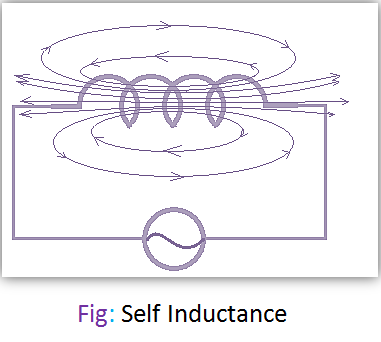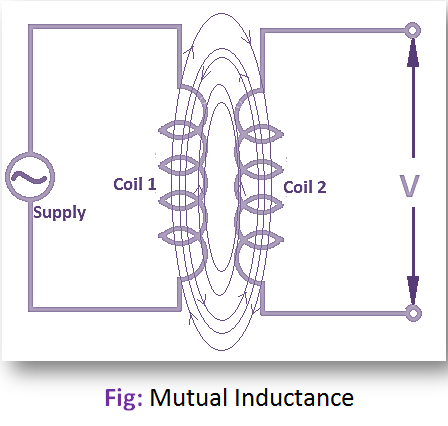# Self Inductance and Mutual Inductance

## Self Inductance:

Let us take a closed coil and the current is supplied to the coil by a source, then it will produce magnetic flux. Now with the variation of current, the flux will also change. This change of flux will produce an emf in the coil. This generated or induced emf is called the Self Inductance.Now the self-inductance (L) of a coil is the characteristic or property of the coil, by which an emf is generated when the current is varied through the coil. It is denoted by ‘L’ and the unit is Henry. It depends upon the shape of the coil used, the square of the number of turns of the coil, and neighboring any magnetic material.

## Mutual Inductance:

Let us take a coil ‘x’ which is placed close to other coil ‘y’. Now if the current of coil ‘x’ can be varied or changed, then an emf will be generated in the coil ‘y’. The generated or induced emf in coil ‘y’ exists so long as the current in coil ‘x’ changes but is not found when the current through coil ‘x’ is steady. This is known as mutual induced emf.The mutual inductance of a coil is the characteristics or property of a coil by which an emf is generated in a coil when there is a variation or change of current through its nearby coil. It is denoted by ‘M’. The unit is Henry. It depends on the closeness of the coil and the magnitude of the variation of current.